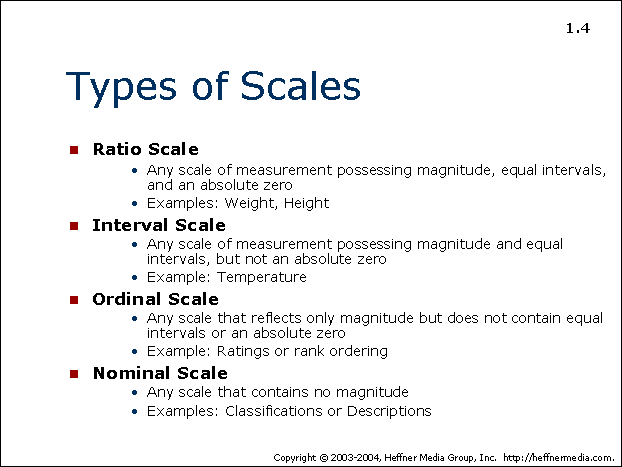# 04: Ratio, Interval, Ordinal, and Nominal Scales## Types of Scales

• Ratio Scale
• Any scale of measurement possessing magnitude, equal intervals, and an absolute zero
• Examples: Weight, Height
• Interval Scale
• Any scale of measurement possessing magnitude and equal intervals, but not an absolute zero
• Example: Temperature
• Ordinal Scale
• Any scale that reflects only magnitude but does not contain equal intervals or an absolute zero
• Example: Ratings or rank ordering
• Nominal Scale
• Any scale that contains no magnitude
• Examples: Classifications or Descriptions